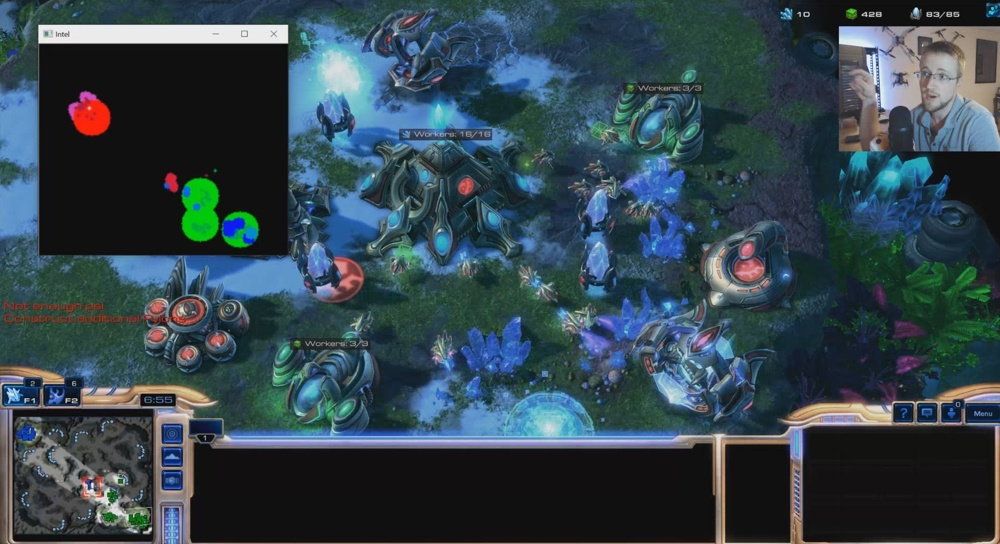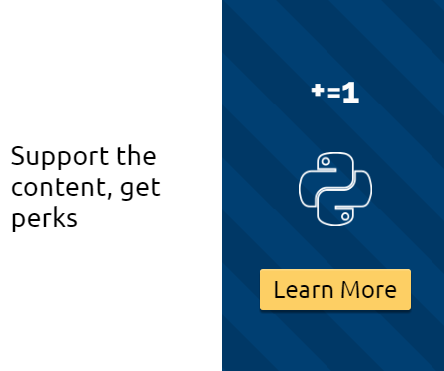## Scouting and more Visual inputs - Python AI in StarCraft II p.8

Welcome to part 8 of the AI in StarCraft II series with Python, where we're now applying deep learning to our AI. In this tutorial, we're going to be working on creating more visualizations for the input to our neural network.

To begin, we want to visualize the rest of our units. In an effort to save space, I have elected to create a `draw_dict`, which contains unit type as the key, and then the values are the size and color of this unit type that I want to draw onto our visualization. So, inside of the `intel` method:

```        draw_dict = {
NEXUS: [15, (0, 255, 0)],
PYLON: [3, (20, 235, 0)],
PROBE: [1, (55, 200, 0)],
ASSIMILATOR: [2, (55, 200, 0)],
GATEWAY: [3, (200, 100, 0)],
CYBERNETICSCORE: [3, (150, 150, 0)],
STARGATE: [5, (255, 0, 0)],
VOIDRAY: [3, (255, 100, 0)],
}```

Now, we can iterate over this with:

```        for unit_type in draw_dict:
pos = unit.position
cv2.circle(game_data, (int(pos), int(pos)), draw_dict[unit_type], draw_dict[unit_type], -1)

```

Next, we want to draw our enemy's buildings and units as well. To do this, I am going to just draw their "main base" (nexus, command center, and hatchery) as the same size as we draw our nexuses, and the rest of them as a size 5. We could get a bit more fancy with this and draw military buildings different from others..etc, just like we're doing with our own buildings. I may take this approach, but, for now, I will just do this. If we find this method to be successful, I will consider other changes like this. So, for enemy buildings:

```        main_base_names = ["nexus", "commandcenter", "hatchery"]
for enemy_building in self.known_enemy_structures:
pos = enemy_building.position
if enemy_building.name.lower() not in main_base_names:
cv2.circle(game_data, (int(pos), int(pos)), 5, (200, 50, 212), -1)
for enemy_building in self.known_enemy_structures:
pos = enemy_building.position
if enemy_building.name.lower() in main_base_names:
cv2.circle(game_data, (int(pos), int(pos)), 15, (0, 0, 255), -1)
```

Now, for the enemy units. We'd like to at least differentiate between the workers and other units. Again, later, we might want to differentiate all the various units and then the network could maybe learn them all. For now, however:

```        for enemy_unit in self.known_enemy_units:

if not enemy_unit.is_structure:
worker_names = ["probe",
"scv",
"drone"]
# if that unit is a PROBE, SCV, or DRONE... it's a worker
pos = enemy_unit.position
if enemy_unit.name.lower() in worker_names:
cv2.circle(game_data, (int(pos), int(pos)), 1, (55, 0, 155), -1)
else:
cv2.circle(game_data, (int(pos), int(pos)), 3, (50, 0, 215), -1)
```

After this, we're going to have a hard time actually seeing enemy buildings and such, except when we're attacking. This isn't really ideal, so it's time we build a scout. Scouts are a bit different per class, but, for Protoss, it's the `observer`. To build the observer, we need a robotics facility. To build that, we need a cybernetics core, which needs a gateway. Luckily, we already have everything we need, except for the `robotics facility`, so let's add that!

To begin, we want to import those units:

```from sc2.constants import NEXUS, PROBE, PYLON, ASSIMILATOR, GATEWAY, \
CYBERNETICSCORE, STALKER, STARGATE, VOIDRAY, OBSERVER, ROBOTICSFACILITY```

Next, in our `offensive_force_buildings` method, we want to add:

```            if self.units(CYBERNETICSCORE).ready.exists:
if len(self.units(ROBOTICSFACILITY)) < 1:
await self.build(ROBOTICSFACILITY, near=pylon)```

The full method now being:

```    async def offensive_force_buildings(self):
#print(self.iteration / self.ITERATIONS_PER_MINUTE)

await self.build(CYBERNETICSCORE, near=pylon)

elif len(self.units(GATEWAY)) < 1:
await self.build(GATEWAY, near=pylon)

if len(self.units(ROBOTICSFACILITY)) < 1:
await self.build(ROBOTICSFACILITY, near=pylon)

if len(self.units(STARGATE)) < (self.iteration / self.ITERATIONS_PER_MINUTE):
await self.build(STARGATE, near=pylon)```

Next, we want to build the scout, and then of course do some scouting. I am going to build and scout all in the same, unique method, since I want the scout to take priority over all else anyway. In our `on_step` method, at the top of all of our methods:

`        await self.scout()`

Now let's create that method:

```    async def scout(self):
if len(self.units(OBSERVER)) > 0:
scout = self.units(OBSERVER)
if scout.is_idle:
enemy_location = self.enemy_start_locations
move_to = self.random_location_variance(enemy_location)
print(move_to)
await self.do(scout.move(move_to))

else:
if self.can_afford(OBSERVER) and self.supply_left > 0:
await self.do(rf.train(OBSERVER))```

So this method checks to see if we have an observer. If we do, and if it is idle, we find the enemy start location, and move to an area that is a slight random variance on that enemy location.

If we don't have an observer, we create one.

Now we just need to make that random variance method:

```    def random_location_variance(self, enemy_start_location):
x = enemy_start_location
y = enemy_start_location

x += ((random.randrange(-20, 20))/100) * enemy_start_location
y += ((random.randrange(-20, 20))/100) * enemy_start_location

if x < 0:
x = 0
if y < 0:
y = 0
if x > self.game_info.map_size:
x = self.game_info.map_size
if y > self.game_info.map_size:
y = self.game_info.map_size

go_to = position.Point2(position.Pointlike((x,y)))
return go_to```

This one isn't too complicated, except for the position itself. You cannot move a unit to just some coordinates, you have to move them to a `position`. To do this, you either create a `Point2` or `Point3` position (depending on whether or not you're passing elevation). Then you need the points to pass to be these `Pointlike` objects instead.

Next, we'll add drawing the robotics facility to our draw dict:

`ROBOTICSFACILITY: [5, (215, 155, 0)],`

Then the last thing we'll actually draw is the observer unit:

```        for obs in self.units(OBSERVER).ready:
pos = obs.position
cv2.circle(game_data, (int(pos), int(pos)), 1, (255, 255, 255), -1)
```

That could cover it, the full code up to this point:

```import sc2
from sc2 import run_game, maps, Race, Difficulty, position
from sc2.player import Bot, Computer
from sc2.constants import NEXUS, PROBE, PYLON, ASSIMILATOR, GATEWAY, \
CYBERNETICSCORE, STALKER, STARGATE, VOIDRAY, OBSERVER, ROBOTICSFACILITY
import random
import cv2
import numpy as np

class SentdeBot(sc2.BotAI):
def __init__(self):
self.ITERATIONS_PER_MINUTE = 165
self.MAX_WORKERS = 50

async def on_step(self, iteration):
self.iteration = iteration
await self.scout()
await self.distribute_workers()
await self.build_workers()
await self.build_pylons()
await self.build_assimilators()
await self.expand()
await self.offensive_force_buildings()
await self.build_offensive_force()
await self.intel()
await self.attack()

def random_location_variance(self, enemy_start_location):
x = enemy_start_location
y = enemy_start_location

x += ((random.randrange(-20, 20))/100) * enemy_start_location
y += ((random.randrange(-20, 20))/100) * enemy_start_location

if x < 0:
x = 0
if y < 0:
y = 0
if x > self.game_info.map_size:
x = self.game_info.map_size
if y > self.game_info.map_size:
y = self.game_info.map_size

go_to = position.Point2(position.Pointlike((x,y)))
return go_to

async def scout(self):
if len(self.units(OBSERVER)) > 0:
scout = self.units(OBSERVER)
if scout.is_idle:
enemy_location = self.enemy_start_locations
move_to = self.random_location_variance(enemy_location)
print(move_to)
await self.do(scout.move(move_to))

else:
if self.can_afford(OBSERVER) and self.supply_left > 0:
await self.do(rf.train(OBSERVER))

async def intel(self):
game_data = np.zeros((self.game_info.map_size, self.game_info.map_size, 3), np.uint8)

# UNIT: [SIZE, (BGR COLOR)]
'''from sc2.constants import NEXUS, PROBE, PYLON, ASSIMILATOR, GATEWAY, \
CYBERNETICSCORE, STARGATE, VOIDRAY'''
draw_dict = {
NEXUS: [15, (0, 255, 0)],
PYLON: [3, (20, 235, 0)],
PROBE: [1, (55, 200, 0)],
ASSIMILATOR: [2, (55, 200, 0)],
GATEWAY: [3, (200, 100, 0)],
CYBERNETICSCORE: [3, (150, 150, 0)],
STARGATE: [5, (255, 0, 0)],
ROBOTICSFACILITY: [5, (215, 155, 0)],

VOIDRAY: [3, (255, 100, 0)],
#OBSERVER: [3, (255, 255, 255)],
}

for unit_type in draw_dict:
pos = unit.position
cv2.circle(game_data, (int(pos), int(pos)), draw_dict[unit_type], draw_dict[unit_type], -1)

main_base_names = ["nexus", "supplydepot", "hatchery"]
for enemy_building in self.known_enemy_structures:
pos = enemy_building.position
if enemy_building.name.lower() not in main_base_names:
cv2.circle(game_data, (int(pos), int(pos)), 5, (200, 50, 212), -1)
for enemy_building in self.known_enemy_structures:
pos = enemy_building.position
if enemy_building.name.lower() in main_base_names:
cv2.circle(game_data, (int(pos), int(pos)), 15, (0, 0, 255), -1)

for enemy_unit in self.known_enemy_units:

if not enemy_unit.is_structure:
worker_names = ["probe",
"scv",
"drone"]
# if that unit is a PROBE, SCV, or DRONE... it's a worker
pos = enemy_unit.position
if enemy_unit.name.lower() in worker_names:
cv2.circle(game_data, (int(pos), int(pos)), 1, (55, 0, 155), -1)
else:
cv2.circle(game_data, (int(pos), int(pos)), 3, (50, 0, 215), -1)

pos = obs.position
cv2.circle(game_data, (int(pos), int(pos)), 1, (255, 255, 255), -1)

# flip horizontally to make our final fix in visual representation:
flipped = cv2.flip(game_data, 0)
resized = cv2.resize(flipped, dsize=None, fx=2, fy=2)

cv2.imshow('Intel', resized)
cv2.waitKey(1)

async def build_workers(self):
if (len(self.units(NEXUS)) * 16) > len(self.units(PROBE)) and len(self.units(PROBE)) < self.MAX_WORKERS:
if self.can_afford(PROBE):
await self.do(nexus.train(PROBE))

async def build_pylons(self):
if self.supply_left < 5 and not self.already_pending(PYLON):
if nexuses.exists:
if self.can_afford(PYLON):
await self.build(PYLON, near=nexuses.first)

async def build_assimilators(self):
vaspenes = self.state.vespene_geyser.closer_than(15.0, nexus)
for vaspene in vaspenes:
if not self.can_afford(ASSIMILATOR):
break
worker = self.select_build_worker(vaspene.position)
if worker is None:
break
if not self.units(ASSIMILATOR).closer_than(1.0, vaspene).exists:
await self.do(worker.build(ASSIMILATOR, vaspene))

async def expand(self):
if self.units(NEXUS).amount < (self.iteration / self.ITERATIONS_PER_MINUTE) and self.can_afford(NEXUS):
await self.expand_now()

async def offensive_force_buildings(self):
#print(self.iteration / self.ITERATIONS_PER_MINUTE)

await self.build(CYBERNETICSCORE, near=pylon)

elif len(self.units(GATEWAY)) < 1:
await self.build(GATEWAY, near=pylon)

if len(self.units(ROBOTICSFACILITY)) < 1:
await self.build(ROBOTICSFACILITY, near=pylon)

if len(self.units(STARGATE)) < (self.iteration / self.ITERATIONS_PER_MINUTE):
await self.build(STARGATE, near=pylon)

async def build_offensive_force(self):
if self.can_afford(VOIDRAY) and self.supply_left > 0:
await self.do(sg.train(VOIDRAY))

def find_target(self, state):
if len(self.known_enemy_units) > 0:
return random.choice(self.known_enemy_units)
elif len(self.known_enemy_structures) > 0:
return random.choice(self.known_enemy_structures)
else:
return self.enemy_start_locations

async def attack(self):
# {UNIT: [n to fight, n to defend]}
aggressive_units = {VOIDRAY: [8, 3]}

for UNIT in aggressive_units:
if self.units(UNIT).amount > aggressive_units[UNIT] and self.units(UNIT).amount > aggressive_units[UNIT]:
for s in self.units(UNIT).idle:
await self.do(s.attack(self.find_target(self.state)))

elif self.units(UNIT).amount > aggressive_units[UNIT]:
if len(self.known_enemy_units) > 0:
for s in self.units(UNIT).idle:
await self.do(s.attack(random.choice(self.known_enemy_units)))

run_game(maps.get("AbyssalReefLE"), [
Bot(Race.Protoss, SentdeBot()),
Computer(Race.Terran, Difficulty.Hard)
], realtime=False)
```

Example of what this produces:Alright, now that we have this, we're ready to add some finishing touches and to begin building our actual initial training dataset!

The next tutorial:• Introduction and Collecting Minerals - Python AI in StarCraft II p.1

• Workers and Pylons - Python AI in StarCraft II p.2

• Geysers and Expanding - Python AI in StarCraft II p.3

• Building an AI Army - Python AI in StarCraft II p.4

• Commanding your AI Army - Python AI in StarCraft II p.5

• Defeating Hard AI - Python AI in StarCraft II p.6

• Deep Learning with SC2 Intro - Python AI in StarCraft II p.7

• Scouting and more Visual inputs - Python AI in StarCraft II p.8
• Building our training data - Python AI in StarCraft II p.9

• Building Neural Network Model - Python AI in StarCraft II p.10

• Training Neural Network Model - Python AI in StarCraft II p.11

• Using Neural Network Model - Python AI in StarCraft II p.12

• Version 2 Changes - Python AI in StarCraft II p.13

• Improving Scouting - Python AI in StarCraft II p.14

• Adding Choices - Python AI in StarCraft II p.15

• Visualization Changes - Python AI in StarCraft II p.16

• More Training and Findings - Python AI in StarCraft II p.17